# Predict mood better than with language alone

Go beyond transcription with multimodal AI for human understanding in audio and videoWhy Hume

### Train and deploy your own expression models to measure any outcome involving human communicationself.__wrap_b=(t,r,e)=>{e=e||document.querySelector(`[data-br="\${t}"]`);let s=e.parentElement,a=T=>e.style.maxWidth=T+"px";e.style.maxWidth="";let n=s.clientWidth,u=s.clientHeight,o=n/2-.25,c=n+.5,p;if(n){for(a(o),o=Math.max(e.scrollWidth,o);o+1<c;)p=Math.round((o+c)/2),a(p),s.clientHeight===u?c=p:o=p;a(c*r+n*(1-r))}e.__wrap_o||(typeof ResizeObserver!="undefined"?(e.__wrap_o=new ResizeObserver(()=>{self.__wrap_b(0,+e.dataset.brr,e)})).observe(s): false&&false)};self.__wrap_b("undefined",1)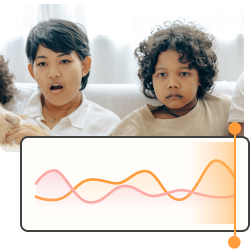###### Facial Expression
Model

Face + Body

Differentiate 37 kinds of facial movement that are recognized as conveying distinct meanings, and the many ways they are blended together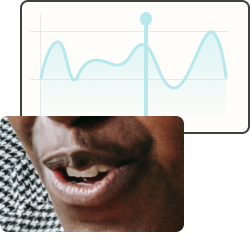###### Speech Prosody
Model

Voice

Discover over 25 patterns of tune, rhythm, and timbre that imbue everyday speech with complex, blended meanings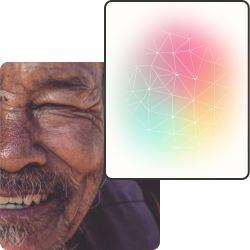###### FACS 2.0
Model

Face + Body

An improved, automated facial action coding system (FACS): measure 26 facial action units (AUs) and 29 other features with even less bias than traditional FACS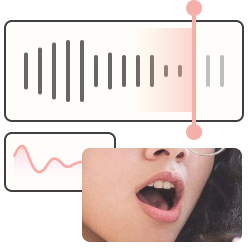###### Vocal Expression
Model

Voice

Differentiate 28 kinds of vocal expression recognized as conveying distinct meanings, and the many ways they are blended together

#### Latest releases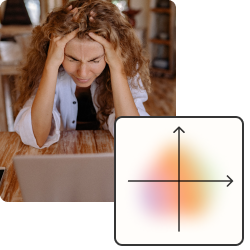###### Valence & Arousal
Model

Multimodal

Predict perceived valence and arousal in facial expression, vocal bursts, speech, or language###### Facial Expression
Model

Face + Body

Differentiate 37 kinds of facial movement that are recognized as conveying distinct meanings, and the many ways they are blended together###### Vocal Call Types
Model

Voice

Explore vocal utterances by inferring probabilities of 67 descriptors, like 'laugh', 'sigh', 'shriek', 'oh', 'ahh', 'mhm', and more

01

03

How it works

### Using Hume’s science-backed expression embedding models and state of the art language modelsself.__wrap_b=(t,r,e)=>{e=e||document.querySelector(`[data-br="\${t}"]`);let s=e.parentElement,a=T=>e.style.maxWidth=T+"px";e.style.maxWidth="";let n=s.clientWidth,u=s.clientHeight,o=n/2-.25,c=n+.5,p;if(n){for(a(o),o=Math.max(e.scrollWidth,o);o+1<c;)p=Math.round((o+c)/2),a(p),s.clientHeight===u?c=p:o=p;a(c*r+n*(1-r))}e.__wrap_o||(typeof ResizeObserver!="undefined"?(e.__wrap_o=new ResizeObserver(()=>{self.__wrap_b(0,+e.dataset.brr,e)})).observe(s): false&&false)};self.__wrap_b("undefined",1)

The Science

No one can read minds, so we start by using statistics to ask what expressions mean to the people making them

Our data-driven science represents real emotional behavior with 3x more precision than traditional approaches

We use scientific control and globally diverse data to remove biases that are entrenched in most AI models

1 of 3

Principles & Testimonial

### Why developers and product managers love building with Humeself.__wrap_b=(t,r,e)=>{e=e||document.querySelector(`[data-br="\${t}"]`);let s=e.parentElement,a=T=>e.style.maxWidth=T+"px";e.style.maxWidth="";let n=s.clientWidth,u=s.clientHeight,o=n/2-.25,c=n+.5,p;if(n){for(a(o),o=Math.max(e.scrollWidth,o);o+1<c;)p=Math.round((o+c)/2),a(p),s.clientHeight===u?c=p:o=p;a(c*r+n*(1-r))}e.__wrap_o||(typeof ResizeObserver!="undefined"?(e.__wrap_o=new ResizeObserver(()=>{self.__wrap_b(0,+e.dataset.brr,e)})).observe(s): false&&false)};self.__wrap_b("undefined",1)

End-to-end infrastructure to make all of your videos searchable

“Hume Ai continually amazes me as to what I can find in all parts of video – be it the video itself, the conversation or text on screen – not only specific results but an understanding of the context of the query. With each new search, I discover new results and possibilities of the technology for our customers."Pedro AlmeidaPnas, CEO

Built for product defining developers

### Build the next, highly-improved generation of multimodal AI-powered productsself.__wrap_b=(t,r,e)=>{e=e||document.querySelector(`[data-br="\${t}"]`);let s=e.parentElement,a=T=>e.style.maxWidth=T+"px";e.style.maxWidth="";let n=s.clientWidth,u=s.clientHeight,o=n/2-.25,c=n+.5,p;if(n){for(a(o),o=Math.max(e.scrollWidth,o);o+1<c;)p=Math.round((o+c)/2),a(p),s.clientHeight===u?c=p:o=p;a(c*r+n*(1-r))}e.__wrap_o||(typeof ResizeObserver!="undefined"?(e.__wrap_o=new ResizeObserver(()=>{self.__wrap_b(0,+e.dataset.brr,e)})).observe(s): false&&false)};self.__wrap_b("undefined",1)

Continuously updated models based on psychologically valid, peer-reviewed, published research

Run

Request

Our Blog

### Latest blog postsself.__wrap_b=(t,r,e)=>{e=e||document.querySelector(`[data-br="\${t}"]`);let s=e.parentElement,a=T=>e.style.maxWidth=T+"px";e.style.maxWidth="";let n=s.clientWidth,u=s.clientHeight,o=n/2-.25,c=n+.5,p;if(n){for(a(o),o=Math.max(e.scrollWidth,o);o+1<c;)p=Math.round((o+c)/2),a(p),s.clientHeight===u?c=p:o=p;a(c*r+n*(1-r))}e.__wrap_o||(typeof ResizeObserver!="undefined"?(e.__wrap_o=new ResizeObserver(()=>{self.__wrap_b(0,+e.dataset.brr,e)})).observe(s): false&&false)};self.__wrap_b("undefined",1)

Our latest product updates, developer news, platform tutorials, and How-To guides.